# How to use the UNICHAR Function in Excel

UNICODE is computing standard for the unified encoding, representation, and handling of text in most of the world's writing systems. The text value used to retrieve the code character. The Unicode character that is returned can be a string of characters, for example in UTF - 8 or UTF - 16 codes

The UNICHAR function is a numerical Unique code of any character in Excel.
Syntax:

=UNICHAR ( number )

number : a valid numerical code for the character
Let’s understand this function using it in an example.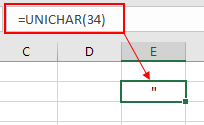As you can see in the above snapshot the UNICHAR function takes the 34 numerical code for the character quotation mark as an argument and returns its defined character which is quotation ( " ) .

Now we will understand it using cell references in the formula.

Here we have a list of characters we need the UNICHAR of
Characters using the function.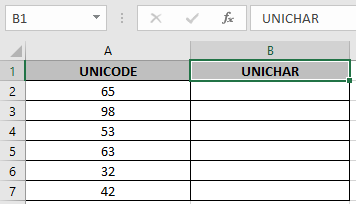Use the formula:

=UNICHAR ( A2 )

A2 : argument provided as cell reference but provides the character for the valid numerical code in the referenced cell.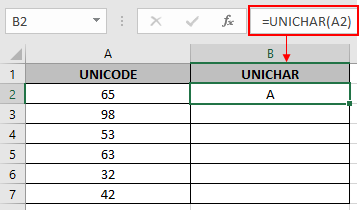Here the argument to the function is given as cell reference.
Copy the formula in other cells using shortcut Ctrl + D.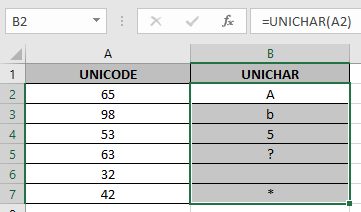The function returns a Value error if empty cell is given as a referenced cell.

You can use the UNICODE function to get the code out of a unique character.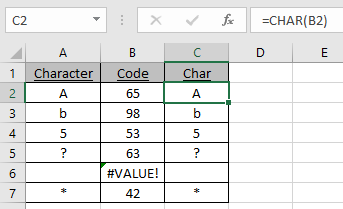As you can see the UNICODE function returns the ANSI CODE whereas UNICHAR function returns the character from the CODE.

Notes:

1. The function returns #VALUE! Error, If the number is invalid, as excel have predefined code for text char.
2. Use the UNICODE function to perform the just exact opposite to UNICHAR function. UNICODE function returns the code for the given input char to the function.
3. Text argument to the function can be given as cell reference or directly.

Hope you understood how to use the UNICHAR function and referring cell in Excel. Explore more articles on Excel TEXT functions here. Please feel free to state your query or feedback for the above article.

Related Articles

How to use the CODE function in Excel

How to use the CHAR function in Excel

How to use Wildcards in Excel

Popular Articles

Edit a dropdown list

If with conditional formatting

If with wildcards

Vlookup by date

Terms and Conditions of use

The applications/code on this site are distributed as is and without warranties or liability. In no event shall the owner of the copyrights, or the authors of the applications/code be liable for any loss of profit, any problems or any damage resulting from the use or evaluation of the applications/code.